## Payment Configuration

Generic selectors
Exact matches only
Search in title
Search in content

# Form Calculations

With the 123FormBuilder online form builder, you can perform calculations on your web forms & surveys. Use form calculations to add mathematical operations between your fields on your online forms.

# Assign Values

First, you need to assign the values to your products/services. To do so, please add a choice field (single/multiple choice or dropdown field) and list the available choices.Click the field to open its options in the left-side panel and select Value / Price. Next to each option type their correspondent price.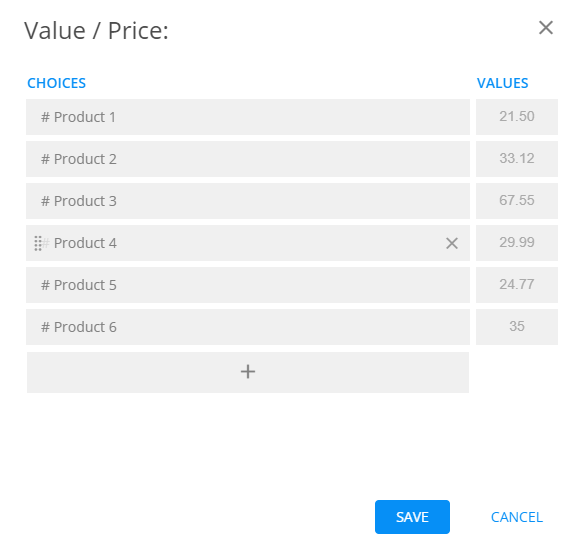If you need the user to type the amount, add a number field and assign the value “1”. By default, a field has no value assigned to it, meaning the input will be multiplied with zero. The form will calculate input value X 1. ( e.g user types 123, the number field will calculate 123 X 1= 123  instead of 123 X 0= 0).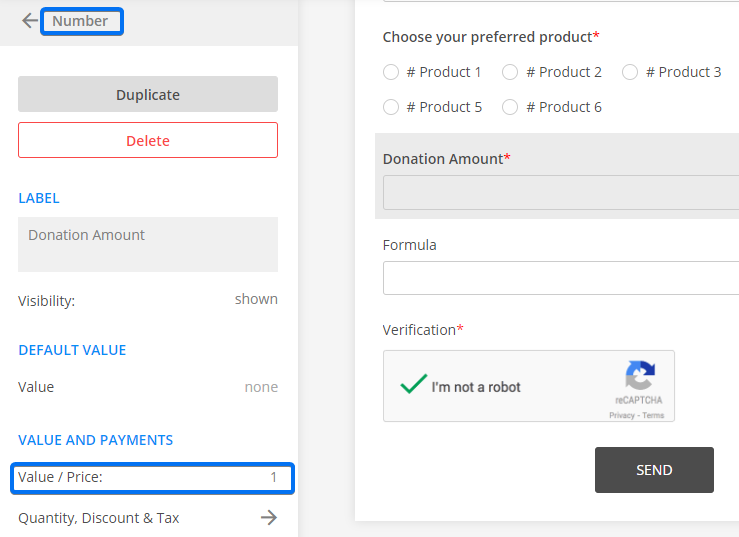When you are using a number (input) field, it works as a quantity field. Assign the full price to the field (e.g \$150). If the user types 2, the calculation will be 2 X 150 = 300\$.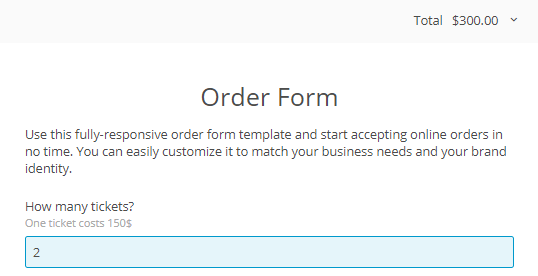# Create formulas

To create mathematical operations on your form, add a formula field on the form. This field will show the total value in real-time.

Click to add a formula field. A calculator will appear where you can add operations between your form fields. Use the Add field button within the calculator to add your form fields. Once the field is selected, it will be automatically added to the formula. You can use your keyboard to add operators and values.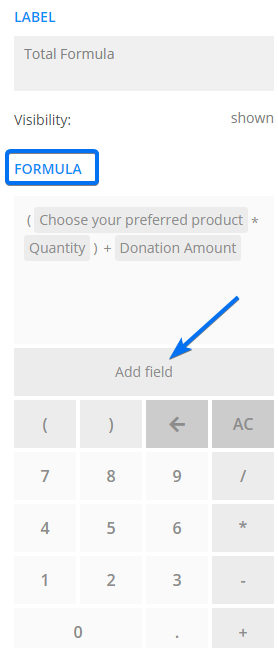You can also add calculations between Date fields. The operation between two Date fields will return the value in the number of days.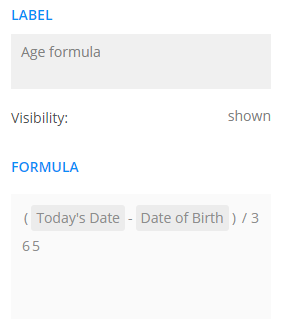• Important Notes
You don’t need to enable payments on your form in order to use form calculations. Formula fields are read-only.

Here how the form configuration used in this guide is calculating.

1.jeet kumar says:

How to make a invoice form which have data list and their prize like ms excel

2.paul dipre says:

how do you round decimal places in formula results

1.Julia says: